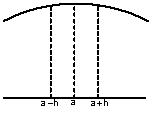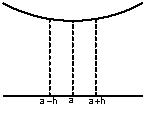Click to Chat

1800-1023-196

+91-120-4616500

CART 0

• 0

MY CART (5)

Use Coupon: CART20 and get 20% off on all online Study Material

ITEM
DETAILS
MRP
DISCOUNT
FINAL PRICE
Total Price: Rs.

There are no items in this cart.
Continue Shopping```Concept of Local Maximum and Local Minimum

Local Maximum

A function f(x) is said to have a local maximum at x=a if the value of

f(a) is greater than all the values of f(x) in a small neighbourhood of x=a.

Mathematically, f (a) > f (a – h) and f (a) > f (a + h) where h > 0, then a is called the point of local maximum.Fig. Local MaximaLocal Minimum

A function f(x) is said to have a local minimum at x = a, if the value of the function at x = a is less than the value of the function at the neighboring points of x = a. Mathematically, f (a) < f (a – h) and f (a) < f (a + h) where h > 0, then a is called the point of local minimum.

A point of local maximum or a local minimum is also called a point of local extremum.Fig. Local Minima

Working Rule to Determine the points of Local Maxima and Local Minima

1. First Derivative Test: If f'(a)  = 0 and f'(x)  changes it’s sign while passing through the point x = a, then

(i) f(x) would have a local maximum at x = a if f'(a–0)  > 0 and  f'(a+0) < 0. It means that f'(x) should change it’s sign from positive to negative e.g. f (x) = –x2 has local maxima at x = 0.

(ii) f(x) would have local minimum at x = a if f'(a–0) < 0  and f'(a+0) > 0 . It means that f'(x) should change it’s sign from negative to positive. e.g. f (x) = x2 has local minima at x = 0.

(iii) If f(x) doesn’t change it’s sign while passing through x = a, then f (x) would have neither a maximum nor minimum at x = a. e.g. f (x) = x3 doesn’t have any local maxima or minima at x = 0.

2. Second Derivative Test:

Step I: Let f(x) be a differentiable function on a given interval and let f’’ be continuous at stationary point. Find f ‘ (x) and solve the equation f ‘ (x) = 0 given let x = a, b, … be solutions.

Step II: Case (i) : If f ‘‘ (a) < 0 then f(a) is maximum. (Refer to above figure)

Case (ii): If f ‘’(a) > 0 then f(a) is minimum. (Refer the above figure)

Note:

(i) If f’’(a) = 0 the second derivatives test fails in that case we have to go back to the first derivative test.

(ii) If f’’(a) = 0 and a is not a point of local maximum nor local minimum then a is a point of inflection.

3. nth Derivative Test: It is nothing but the general version of the second derivative test. It says that if,

f' (a) = f'(a) = f' (a) =………. = f' (a) = 0 and fn+1 (a) ¹ 0 (all derivatives of the function up to order ‘n’ vanishes and (n + 1)th order derivative does not vanish at x = a), then f (x) would have a local maximum or minimum at x = a iff n is odd natural number and that x = a would be a point of local maxima if fn+1 (a) < 0 and would be a point of local minima if fn+1 (a) > 0.

It is clear that the last two tests are basically the mathematical representation of the first derivative test. But that shouldn’t diminish the importance of these tests. Because at time it’s becomes very difficult to decide whether f'(x) changes it’s sign or not while passing through point x = a, and the remaining tests may come handy in these type of situations.

Illustration 14: Find the local maximum and local minimum for the following function :

(i) f(x) = 2x3 – 21x + 36x – 20

(ii) f(x) = – x + 2sinx,  0 < x × < 2θ

Solution :  (i) f(x) = 2x3  – 21x2 + 36x – 20

f'(x) = 3x2 – 12x + 9

For local max. and local min. f'(x) = 0; k3x2 – 12x + 9 = 0

or         3(x2 – 4x + 3) = 0 or x2 – 4x + 3 = 0

or         (x–1) (x–3) = 0,              x = 1, x = 3

f"(x) = 6x – 12

at x = 1, f"(x) = 6 × 1 – 12 = – 6 < 0 negative

at x = 3, f"(x) = 6 × 3 – 12 = – 6 > 0 positive

at x = 1, f(x) has maximum,

f(1) = 1 – 6 + 9 + 7 = 11

at x =3, f(x) has minimum,

f(3) = 27 – 54 + 27 + 7 = 7

(ii)         f(x) = 2x3 – 21x2 + 36x – 20

f'(x) = 6x2 – 42x + 36 = 6 (x2 – 7x + 6)

= 6(x–1)(x–6)

For local max. and local min.

f'(x) = 0 => 6(x–1) (x–6) = 0

x = 16

f"(x) = 12x – 42 = 6 (2x – 7)

f"(1) = 6 (2–7) = –30 < 0

f"(6) = 6 (12–7) = –30 < 0

at x = 1 f (x) has local max and at x = 6 there is local min,

Local max value = f(1) = 2(1)3 – 21(1)2 + 36(1) – 20

= 2 – 21 + 36 – 20 = –3

Local min value = f(6) = 2(216) – 21(36) + 36(6) – 20

= 432 – 756 + 216 – 20 = –128

(iii) f(x) = – x + 2 sin x

f'(x) = –1 + 2 sin x

For local max. and local min. f'(x) = 0

–1 + 2 cos x = 0       or        cos x = 1/2

= cos π/3 = cos (2/π – π/3) = cos 5π/3

x = θ/3,  5θ/3

f"(x) = – 2sin x

∴ f"(π/3) = –2 sin π/3 = –2(√3/2) = –√3 < 0

∴ f"(5π/3) = –2 sin 5π/3 = –2(–√3/2) = √3 < 0

So at x = θ/3 the function has local max and its value is

= f(π/3) = – π/3 + 2 sin π/3

= –π/3 + 2 × √3/2 = –π/3 + /3

So at x = 5π/3 the function has local min and its value is

= f(5π/3) = – 5π/3 + 2 sin 5π/3

= –5π/3 – 2sin π/3 = – 5π/3 – 2 × √3/2

= – 5π/3 – √3

Illustration 15: If f(x) = |4x – x2 – 3| where x ∈ then find the points of local maxima and minima.

Solution: Clearly, x = 1, 3 are the points of local minima and x = 2 is a local maxima.

To read more, Buy study materials of Applications of Derivatives comprising study notes, revision notes, video lectures, previous year solved questions etc. Also browse for more study materials on Mathematics here.
```### Course Features

• 731 Video Lectures
• Revision Notes
• Previous Year Papers
• Mind Map
• Study Planner
• NCERT Solutions
• Discussion Forum
• Test paper with Video Solution